Edition Date: June 2018
Part Number: 370858P-01
View Product InfoDIAdem 2017 HelpDIAdem 2018 HelpDIAdem 2019 HelpDIAdem 2020 HelpDIAdem 2020 SP1 Help

Use DIAdem ANALYSIS to execute mathematical data analyses. DIAdem has the following function groups: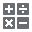Use Basic Mathematics to perform basic mathematical calculations.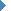Further information

• Subtract: Subtracts channels.

• Multiply: Multiplies channels.

• Divide: Divides channels.

• Round: Rounds the channel values.

• Remove Duplicates: Removes multiple channel values.

• Scale: Scales channels.

• Offset Correction: Corrects the channel values by an offset.

• Calculate Reciprocal: Calculates the reciprocal value of channels.

• Normalize: Divides all channel values by the highest absolute value in the data channel.

• Relativize: Converts the single values in a channel into a percentage of the sum of all the values in the channel.

• Calculate Differences: Calculates the difference between two successive single values of a channel.

• Summation: Specifies the running sum of the single values of a channel.

• Differentiate: Differentiates a signal numerically.

• Integrate: Integrates a signal numerically.

• Calculate RMS: Calculates the floating square integral mean of a signal.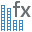Use the Channel Functions to create channels, to convert channels, and to analyze channels.Further information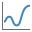Use Curve Fitting, for example, to smooth curves, to approximate curves, and to approximate curves using splines.Further information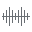Use Signal Analysis, for example, to run fast Fourier transforms (FFT) and order analyses.Further information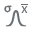Use Statistics to calculate characteristic statistic values and run classifications.Further information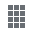Use 3D Basic Functions to convert, transpose, and sort matrices. The basic 3D function is in the 3D menu.Further information

• Create Submatrix: Creates a submatrix from a matrix.

• Append Matrix: Appends a matrix to an existing matrix.

• Convert Matrix: Converts three-dimensional data from a matrix structure into a triplet structure, and vice versa.

• Transpose Matrix: Transposes rows and columns in a matrix.

• Sort Matrix: Monotone increased sorting of the x and y-channels in a matrix with the respective displacement of the z-values.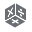Use 3D Arithmetic to calculate matrices, vectors, and scalars; to normalize, relativize, and integrate matrices; and to calculate sums and extreme values of matrices. The 3D arithmetic functions are in the 3D menu.Further information

• Matrix-Matrix Operations: Arithmetically calculates two matrices.

• Matrix-Vector Operations: Multiplies a matrix by a vector, algebraically or componentwise.

• Matrix-Scalar Operations: Adds a constant to a matrix or multiplies a constant by a matrix.

• Normalize: Normalizes the elements of a matrix to the maximum value one.

• Relativize: Calculates the percentage of the total sum of all the matrix elements, for each element in a matrix.

• Summation: Adds up the values in a matrix, in rows or columns.

• Integrate: Calculates the space under a surface that is given through a matrix.

• Calculate Extreme Values: Defines the minimum values and the maximum values of a matrix.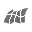Use Surfaces to interpolate and to approximate 3D data, or to calculate contours and the envelope. The surface functions are in the 3D menu.Further information

• Interpolate: Interpolates three-dimensional data with a surface, which runs through the specified points.

• Approximate: Approximates three-dimensional data with a surface, which minimizes the distance to the specified points.

• Calculate Contour Lines: Calculates curves of the same height on a surface.

• Calculate Hull: Calculates convex or non-convex hulls for a set of points.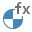Use Crash Analysis to run calculations in the vehicle safety area.Further information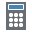Use the Calculator to enter and calculate a formula. In the formula, you can link channels according to their function, request values from variables, and assign values to variables. You can run numeric, Boolean, and text operations in the formula.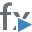Use Execute Calculations to execute predefined calculations.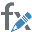Use the Calculation Manager to create calculations, to manage calculations, and to repeat calculations.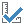Enable the Calculate Quantity-Based button, to include all units of the Units catalog in the calculation. To execute the calculation with the numeric values without including units, disable the Calculate Quantity-Based button.